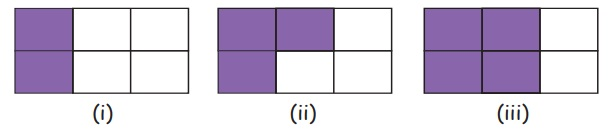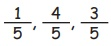Home | | Maths 5th Std | Like fractions and unlike fractions

# Like fractions and unlike fractions

Converting unlike fractions into like fractions

Like fractions and unlike fractions

Consider the following figures:The fraction represented by (i) is 2/6. The fraction represented by

(ii) is 3/6. The fraction represented by (iii) is 4/6

In 2/6, 3/6 and 4/6 , the denominators are the same. Such fractions are called as like fractions.

Like fractions

Fractions with same denominators are called like fractions.

Examples 1/5, 4/5, 3/5Unlike fractions

Fractions with different denominators are called unlike fractions.

Examples: 1/7, 2/9, 9/11Converting unlike fractions into like fractions

To convert unlike fractions into like fractions, we change the denominators of the given fractions into a common denominator. This can be done by finding the common multiples of the denominator.

Example 6.6

Convert 5/6 and 7/9 into like fractions.

Solution

Find common multiples for the numbers 6 and 9

Multiples of 6 : 6,12,18,24,30,36,…

Multiples of 9 : 9,18,27,36,45,…..

Here, the number 18 is a multiple of both 6 and 9 and so 18 is the first common denominator of both the fractions.Thus 15/18 and 14/18 are like fractions, repectively equivalent to 5/6 and 7/9

Example 6.7

Covert 4/8 and 5/16 into like fractions.

Solution

As 16 is twice 8 it is easy to make 16 as the common denominatorThus 8/16 and 15/16 are the required like fractions.

Example 6.8

Find a common denominator for 2/5 and 3/7

Solution

The number 35 is a common multiple of both 7 and 5.

So, take 35 as the common denominator.Therefore 14/35 and 15/35 are the required like fractions.

Tags : Fractions | Term 3 Chapter 6 | 5th Maths , 5th Maths : Term 3 Unit 6 : Fractions
Study Material, Lecturing Notes, Assignment, Reference, Wiki description explanation, brief detail
5th Maths : Term 3 Unit 6 : Fractions : Like fractions and unlike fractions | Fractions | Term 3 Chapter 6 | 5th Maths